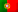Home > Technology > Computer Science > Programming > C++ > Doubly linked lists in C++ (Part I)

# Doubly linked lists in C++ (Part I)

The main difference between singly linked lists, that only have one pointer for the next node, making only possible to iterate the values a single way, and the doubly linked lists is that these last ones have, for each node, two pointers. One pointer for the next node and another pointer for the previous node. This way, it is possible to access both next and previous nodes from any node, thus allowing us to iterate a doubly linked lists in the two directions. Another difference is that, while in a singly linked list we have just a pointer for the “head” of the list, in a doubly linked list we also have a pointer for the “tail” of the list.

Imagine that we have a singly linked list and we are positioned in some node and we need to access the previous node. The only way to do it is by iterating the list all over again, from the beginning, until we reach that node. With a doubly linked list, we only need to use the pointer for the previous node to reach it, which is a great advantage.

But it isn’t always that perfect and these lists have a cost, because for each node we will need some extra bytes to store that “previous node” pointer (4 bytes of a 32 bits machine or 8 bytes for a 64 bits machine), thus consuming more memory.

Now, let’s see the following figure to help us understanding the concept of doubly linked lists.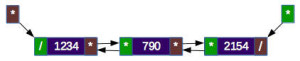In this figure we have a list with 3 nodes. In the red boxes, we have the head pointing to the first node and, for each node, a pointer for the next node, where in the last one this pointer will be set to NULL (end of the list, represented by the slash “/”). And in the green boxes, we have the tail pointing to the last node and, for each node, a pointer for the previous node, where in the first one this pointer will be set to NULL (beginning of the list, also represented by the slash “/”).

To start with, let’s use a simple “struct” to represent our node and let’s name that “struct” by “DNode”. The node will store an integer value:

```struct DNode {
int value;
DNode* next;
DNode* previous;
};
```

So we have an integer variable called “value” and two pointers: “next” and “previous”.

Now, let’s declare the pointers we are going to use in our program in the “main” function:

```    DNode* dnode;
DNode* tail = NULL;
```

Where the pointer “dnode” will be a pointer that we will use in the operations we will perform on the list, the pointer “head” that will point to the first node and the pointer “tail” that will point to the last node.

In the article about singly linked lists, we saw how to list all the values from the beginning of the list. For the moment, I’m just going to give an example on how to list all the values in the list from its end (in reverse order).

```    if (tail==NULL) {
cout << "The list is empty!" << endl;
}
else {
dnode = tail;
while (dnode!=NULL) {
cout << "Value: " << dnode->value << endl;
dnode = dnode->previous;
}
}
```

In this example, we start by verifying if the list is empty by checking if the tail is NULL (line 1). Notice that, we can test the head or the tail, because every time a list is empty, both this pointers shall be pointing to NULL. And by the way, if the list only has one node, it will be pointed by both the head and the tail.

If we have values, then we will start the from the tail (line 5) and we execute a loop until we reach the beginning of the list (line 6), where the pointer “previous” will be pointing to NULL. And since we are reading the list from the end to the beginning, we have to move backwards by using the pointer “previous” (line 8).

Let’s now see how to insert nodes on a doubly linked list.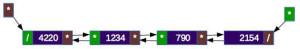In the picture above, we are inserting a node, with the value 4220, at the head of the list. This process is very similar to the insertion at the head on a doubly linked list. However, we need to pay attention to the pointer for the previous node!  In the other previous picture, we saw that the first node had the value “1234”. So, we need to copy the pointer “head” to the pointer “next” of the new node (the one with the value 4220) in order to have it pointing to the node “1234” and change the head for the new node. But, because we have a doubly linked list, we also need to access to the node “1234”, that now it will become the second node, and change it’s pointer “previous” (that was pointing to NULL) to point to the new node. Lastly, on the new node, we need to set its pointer “previous” to NULL (to tell it will be at the beginning of the list).

Let’s see how to perform this operation in C++. We also need to test first if the list is empty, because if it is we will be inserting a first node on the list:

```    dnode = (DNode*) malloc(sizeof(DNode));
dnode->value=4220;
dnode->previous = NULL;
dnode->next = NULL;
tail = dnode;
}
else {
}
```

For both cases, the new node will have its pointer “previous” set to NULL (line 3), because we are at the beginning of the list. Now, notice that, in line 4, we are testing if the head is NULL – which means that list list is empty – and, in this case, the pointer “next” of the new node will need to be NULL, because this will be the first and the last node (line 5) and, therefore, we also need to set the tail to point to this new node (line 6).

If the list is not empty, and following the example represented in the previous picture, the node “1234”, that will become the second node but it’s still being pointed by the head, shall have its pointer “previous” pointing to the new node (line 9) and, in a similar way to the singly linked list, we copy the pointer “head” to the pointer “next” of the new node (line 10).

Finally, on line 12, the head will now be pointing to the new node.

### Inserting at the tail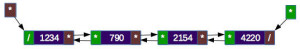In the case of a insertion at the tail, the operation here will be easier than on singly linked lists, where we needed to iterate the entire list in order to get the last node. But since we have now a doubly linked list, we have the pointer “tail” pointing to the last node. In the picture above we are inserting a node with the value 4220 at the tail of the list. In a reverse way of the insertion at the head, we need to copy the pointer “tail” to the pointer “previous” of the new node (“4220”), so we will have it pointing to the node “2154”, and change the pointer “tail” to point to the new node. Once again, and because we have a doubly linked list, we will have to change the pointer “next” of the node “2154”, that will become the penultimate node, to the new node. Finally, in the new node will need to have its pointer “next” set to NULL, since we are at the end of the list.

Let’s now see how the code in C++ will look like. We also need to check if the list is empty!

```    dnode = (DNode*) malloc(sizeof(DNode));
dnode->value=4220;
dnode->next = NULL;
if (tail==NULL) {
dnode->previous = NULL;
}
else {
tail->next = dnode;
dnode->previous = tail;
}
tail = dnode;
```

For both cases, the pointer “next” of the new node will be always NULL, because we are at the end of the list (line 3). In line 4, we also need to test if the list is empty (let me remember you that we can perform this test by using the head or the tail), and if it is empty, the pointer “previous” of the new node shall be set to NULL, since it will be the last and the first node (line 5) and, therefore, the pointer “head” will also need to be pointing to the new node (line 6).

If the list is not empty, and following again the example in the picture, the node “2154”, that will become the penultimate node, but is still being pointed by the head, shall have its pointer “next” pointing to the new node (line 9) and we need to copy the pointer “tail” for the pointer “previous” of the new node (line 10).

Finally, in line 12, the tail will now be pointing to the new node.

### Inserting at the middle of the list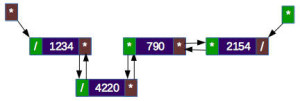Regarding the insertion at the middle of the list, let’s consider again the example in the picture above, where we want to insert a new node, with the value “4220” between the first node (“1234”) and the second node (“790”). As we saw in the singly linked lists, we can access to the node prior to the position where we want to insert the new node, or, if we decide to make the search from the end of the list, we will need to access to the node that follows that position. I will explain how to perform the search by the beginning of the list, but the other way is quite similar. However, remember that we will also need to access to the node that follows the position where we want to insert the new node (in the example, the node “790”), since we need to change its pointer “previous”, as I’m going to explain below.

So, after we reach the node prior to the position where we want to insert the new node, we copy the pointer “next” of that node (that in the picture has the value 1234) to the new node, so it will now be pointing to the next node “790”) and we change the pointer “next” of the node “1234” to point to the new node. Again, as we have a doubly linked list, we also need to access the node “790” and change its pointer “previous” (that was pointing to “1234”) so it will now be pointing to the new node. And, in the new node (“4220”) we will need to change its pointer “previous” to point to the node “1234”, thus keeping the linkage in both directions.

By now, I’m not going to show the code for this case, because it will be used in a function to insert values in a ordered list in the third part of this article. But as a suggestion, I invite the reader to try to write this code!

In the second part we will see how to remove nodes and I’ll give you a complete example that will insert new nodes at the head and at the tail, list the values of the nodes and removes a node with a given value.